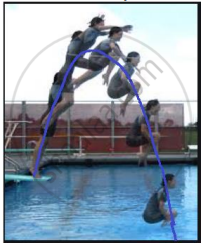# The zeroes of the polynomial r(t) = -12t2 + (k - 3)t + 48 are negative of each other. Then k is ______. - Mathematics

MCQ
Fill in the Blanks

Case Study -1The figure given alongside shows the path of a diver, when she takes a jump from the diving board. Clearly it is a parabola.

Annie was standing on a diving board, 48 feet above the water level. She took a dive into the pool. Her height (in feet) above the water level at any time ‘t’ in seconds is given by the polynomial h(t) such that h(t) = -16t2 + 8t + k.

The zeroes of the polynomial r(t) = -12t2 + (k - 3)t + 48 are negative of each other. Then k is ______.

• 3

• 0

• -1.5

• -3

#### Solution

The zeroes of the polynomial r(t) = -12t2 + (k - 3)t + 48 are negative of each other. Then k is 3.

Explanation:-

When the zeroes are negative of each other, sum of the zeroes = 0

So, (-b)/a=0

(-(k-3))/-12=0

(k-3)/12=0

k - 3 = 0,

i.e. k = 3

Concept: Relationship Between Zeroes and Coefficients of a Polynomial
Is there an error in this question or solution?
Share